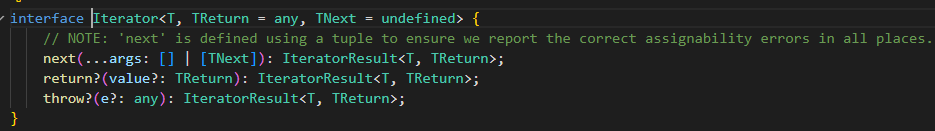# JavaScript – Iterator

## 前言

es6 以后经常可以看到 for...of 的踪迹. 如果你细看会发现它挺神奇的. 不只是 Array 可以被 for...of, 有些对象也可以被 for...of.

const array = [1, 2, 3];
console.log(Array.isArray(array)); // true
for (const value of array) {
console.log(value); // 1, 2, 3
}

const notArray = document.querySelectorAll('h1');
console.log(Array.isArray(notArray)); // false
for (const value of notArray) {
console.log(value); // h1
}

## Iterable 对象

interface Iterable<T> {
[Symbol.Iterator](): Iterator<T>;
}

## Iterator

Iterable 对象的 Symbol.Iterator 方法必须返回一个 iterator.## How to use a Iterator

const Iterator: Iterator<string> = {
next() {
return { value: '1', done: true };
},
};
const { value, done } = iterator.next();
console.log(value); // '1'
console.log(done); // true

next 方法返回一个 value 和一个 boolean done 表示遍历是否完结了.

1. Iterator 是对象, 有一个 next 方法

2. 调用 next 可以拿到一个值, 同时可以知道是否遍历结束. 如果还没有结束意味着可以继续 next 拿到下一个值.

3. Iterator 内部如何实现遍历, 调用者是不知道的, 可能内部是一个 array 或者是一个 formula. 消费者不知道, 也不需要知道. 只要 call next 就对了.

## for...of

const stringList: Iterable<string> & { value: string } = {
value: 'Derrick',
[Symbol.Iterator]() {
let index = 0;
return {
next: () => ({
value: this.value[index++],
done: index === this.value.length + 1,
}),
};
},
};

for (const value of stringList) {
console.log('value', value); // d e r r i c k
}

stringList.value = 'super';

for (const value of stringList) {
console.log('value', value); // s u p e r
}

stringList 是一个 Iterable, 一个拥有 Symbol.Iterator 方法的对象

Symbol.Iterator 返回一个 Iterator, 一个拥有 next 方法的对象. next 返回一个值和 done (表示是否遍历结束)

for...of 开始时, 会调用 Symbol.Iterator 方法得到 stringList 的 Iterator

## Break and ReturnIterator 除了 next 方法, 还可以实现 return 和 throw 方法. throw 是配合 Generator 函数才会用到, 这篇先不提.

return 的用意是, 当调用者中断遍历时通知 iterator. 为了让 Iterator 内部做一些释放内存之类的事情.

for...of 内的 break 语句就会调用 iterator.return (注意: 只有 break 才是中断遍历, continue 并不是哦)

const stringList: Iterable<string> & { value: string } = {
value: 'Derrick',
[Symbol.Iterator]() {
let index = 0;
return {
next: () => ({
value: this.value[index++],
done: index === this.value.length + 1,
}),
return: (value) => {
console.log('return', value);
return {
value: '',
done: true,
};
},
};
},
};

for (const value of stringList) {
if (value === 'i') {
console.log('calling break');
break;
}
console.log('value', value); // d e r r i calling break return
}

const values = [...stringList]; // ['D', 'e', 'r', 'r', 'i', 'c', 'k']